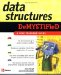# Quiz

 1 What is a queue?2 What is the relationship between a queue and its underlying array?3 Explain how the index of the front and back of the queue is calculated.4 What is the purpose of the enqueue process?5 What is the purpose of the dequeue process?6 Why is the isFull() member method called?7 Why is the isEmpty() member method called?8 What happens to the data stored on the array when the data is removed from the queue?9 What is the purpose of setting the default size of the queue?10 Why does the C++ version of the queue delete the underlying array from memory using the destructor and the Java version of the queue does not?1 A queue is an organization of data where data is stored at the back of the queue and removed from the front of the queue using the first in, first out method. 2 Data stored in a queue is actually stored in an array. The queue tracks which array element is at the front of the queue and which array element is at the back of the queue. 3 The index of the front and back of the queue is calculated by modulus division. First, the value of either the front or back attribute is incremented depending on whether you are calculating the index of the front of the queue or the index of the back of the queue. The result is then divided by the size of the queue using modulus division. The remainder is the index of the array element that is either the front or the back of the queue, depending on which you are calculating. 4 The enqueue process places data at the back of the queue. 5 The dequeue process removes data from the front of the queue. 6 The isFull() member method is called within the enqueue process to determine if there is room to place another item in the queue. 7 The isEmpty() member method is called within the dequeue process to determine if there is an item in the queue to be removed. 8 Removing data from the queue does not remove data from the underlying array. The data remains in the array after the data is removed from the queue. 9 The default size of the queue prevents an error should the programmer forget to pass the size of the queue to the constructor of the Queue class. 10 The destructor in the C++ version of this program removes the underlying array from memory once the instance of the Queue class goes out of scope. Java doesn t have a destructor. Instead, Java has a garbage collector that automatically removes the underlying array from memory some time after the instance of the Queue class goes out of scope. Therefore, there is no need to explicitly remove the array from memory in the Java version of this program.Data Structures Demystified (Demystified)
ISBN: 0072253592
EAN: 2147483647
Year: 2006
Pages: 90

Similar book on Amazon